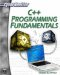# Using the Standard Namespace

Namespaces allow C++ programmers to group a set of global objects or functions under a single name. The general format of a namespaces is the following.

`namespace identifier {  namespace-body }`

The word identifier represents any valid identifier and namespace-body is the set of objects or functions that is included within the namespace. An example follows.

`namespace mynamespace {  int a, b; } `

In the code segment shown here, a and b are normal variables integrated within the namespace named mynamespace. To access these variables from outside the namespace we have to use the scope operator ::. For example, to access the previous variables we would have to put:

`mynamespace::a mynamespace::b `

The use of namespaces is particularly handy in cases where there is a possibility that a global object or function can have the same name as some other object or function. Using namespaces allows you to specify which one you are referring to. Let’s look at a code segment that shows how namespaces can help with cases where two variables have the same name.

`#include <iostream> using std::cout; using std::endl; namespace nameone {  int myvar = 5; } namespace nametwo {  float myvar = 9.6f; } int main () { cout << nameone::myvar << endl; cout << nametwo::myvar << endl;   return 0; }`
 Hint! After the 9.6, there is a small lowercase letter f. This is to denote that this number is a float. If you do not put that there, many C++ compilers will assume that it is a double.

Without the use of namespaces you would get an error trying to use two variables with the same name.

The most common use of namespaces, is by far with the standard namespace. When you include iostream you have been identifying each item you wish use. However, you don’t have to do that if you simply specify that you are using the standard namespace. The following is code snippet that includes the standard namespace.

`#include <iostream>  using namespace std;`

You use namespaces by simply executing the following statement.

`using namespace namespacename;`

Now you can access the elements contained in that namespace simply by calling their names. It’s a little like using the include statements we have already seen in this chapter.

`   // using namespace example    #include <iostream>    namespace nameone    { int myvar = 5;    }    namespace nametwo   { float myvar = 9.6f;   }   int main ()    { using namespace nametwo; cout << myvar << endl; return 0;   }`

In this case, because you stated explicitly that you were using namespace nametwo, whenever you reference the variable myvar, it will be the version contained in namespace nametwo.

You may be asking what this has to do with console input and output, and that’s a good question. This topic is covered here because the standard namespace is what allows you to use names such as cout, cin , and endl. Visual C++ will allow you to use the .h extensions and not include the standard namespace. However this is not standard and not widely supported, so you will normally need to include the namespace. In fact, it’s best to just use the ANSI/ISO standards wherever possible because these standards are supported by ALL C++ compilers. This book uses only ANSI/ISO standard C++, with a few minor exceptions, which are noted in the text. With all of that said, you should plan on using the ANSI/ISO standard way of including the iostream header file.

`#include <iostream> using namespace std ;`C++ Programming Fundamentals (Cyberrookies)
ISBN: 1584502371
EAN: 2147483647
Year: 2005
Pages: 197
Authors: Chuck Easttom